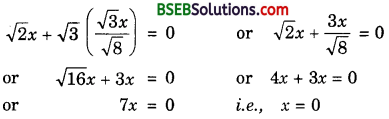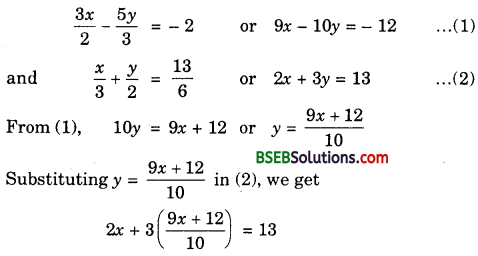# Bihar Board Class 10th Maths Solutions Chapter 3 Pair of Linear Equations in Two Variables Ex 3.3

Bihar Board Class 10th Maths Solutions Chapter 3 Pair of Linear Equations in Two Variables Ex 3.3 Textbook Questions and Answers.

## BSEB Bihar Board Class 10th Maths Solutions Chapter 3 Pair of Linear Equations in Two Variables Ex 3.3

Question 1.
Solve the following pair of linear equations by the substitution method:
1. x + y = 14
x – y = 4

2. s – t = 3
$$\frac{s}{3}$$ + $$\frac{t}{2}$$ = 6

3. 3x – y = 3
9x – 3y = 9

4. 0.2x + 0.3y = 1.3
0.4x + 0.5y = 2.3

5. $$\sqrt{2x}$$ + $$\sqrt{3y}$$ = 0
$$\sqrt{3}$$x – $$\sqrt{8}$$y = 0

6.m $$\frac{3x}{2}$$ – $$\frac{5y}{3}$$ = – 2
$$\frac{x}{3}$$ + $$\frac{y}{2}$$ = $$\frac{13}{6}$$
Solution:
1. The given system of equations is
x + y = 14 …………………. (1)
and x – y = 4 ……………… (2)
From (1), y = 14 – x
Substituting y = 14 – x in (2), we get
x – (14 – x) = 14 or x – 14 + x = 4 or 2x = 4 + 14 or 2x = 18
or x = 9
Putting x = 9 in (1), we get
9 + y = 14 or y = 14 – 9 or y = 5
Hence, the solution of the given system of equations is
x = 9, y = 5

2. The given system of equations is
s – t = 3 …………… (1)
$$\frac{s}{3}$$ + $$\frac{t}{2}$$ = 6
From (1), s = 3 + t
Substituting s = 3 + t in (2), we have
$$\frac{3+t}{3}$$ + $$\frac{t}{2}$$ = 6 or 2(3 + t) + 3t = 36
or 6 + 2t + 3t = 36 or 5t = 30 or t = 6
Putting t = 6 in (1), we get
s – 6 = 3 or s = 3 + 6 = 9
Hence, the solution of the given system of equations is
s = 9, t = 6

3. The given system of equations is
3x – y = 3 …………. (1)
and 9x – 3y = 9 …………… (2)
From (1), y = 3x – 3
Substituting y = 3x – 3 in (2), we get
9x – 3(3x – 3) = 9 or 9x – 9x + 9 = 9
or 9 = 9
This statement is true for all values of x. However, we do not get a specific value of x as a solution. So, we cannot obtain a specific value of y. This situation has arisen because both the given equations are the same.
∴ Equations (1) and (2) have infinitely many solutions.

4. The given system of equations is
0.2x + 0.3y = 1.3 ⇒ 2x + 3y = 13 …………….. (1)
and 0.4x + 0.5y = 2.3 ⇒ 4x + 5y = 23 ……………….. (2)
From (2), 5y = 23 – 4x or y = $$\frac{23-4x}{5}$$
Substituting y = $$\frac{23-4x}{5}$$ in (1), we get
2x + 3($$\frac{23-4x}{5}$$) = 13 or 10x + 69 – 12x = 65
or – 2x = – 4 or x = 2
Putting x = 2 in (1), we get
2 × 2 + 3y = 13 or 3y = 13 – 4 = 9
or y = $$\frac{9}{3}$$ = 3
Hence, the solution of the given system of equations is
x = 2, y = 3

5. The given system of equations is
$$\sqrt{2x}$$ + $$\sqrt{3y}$$ = 0 ………………. (1)
and $$\sqrt{3x}$$ – $$\sqrt{8y}$$ = 0 ………………… (2)
From (2), $$\sqrt{8y}$$ = $$\sqrt{3x}$$ or y = $$\frac{\sqrt{3} x}{\sqrt{8}}$$
Substituting y = $$\frac{\sqrt{3} x}{\sqrt{8}}$$ in (1), we getPutting x = 0 in (1), we get
0 + $$\sqrt{3y}$$ = 0 or y = 0

6. The given system of equations isor 20x + 27x + 36 = 130
or 47x = 130 – 36 or 47x = 94
or x = $$\frac{94}{47}$$ = 2
Putting x = 2 in (1), we get
9 × 2 – 10y = – 12 or – 10y = – 12 – 18
or – 10y = – 30 or y = $$\frac{-30}{-10}$$ = 3
Hence, the solution of the given system of equations is
x = 2, y = 3Question 2.
Solve 2x + 3y = 11 and 2x – 4y = – 24 and hence find the value of ‘m’ for which y = mx + 3.
Solution:
The given system of equations is
2x + 3y = 11 ……………… (1)
and 2x – 4y = – 24 ……………….. (2)
From (1), 3y = 11 – 2x or y = $$\frac{11-2x}{3}$$
Substituting y = $$\frac{11-2x}{3}$$ in (2), we get
2x – 4($$\frac{11-2x}{3}$$) = – 24 or 6x – 44 + 8x = – 72
or 14x = – 72 + 44 or 14x = – 28
or x = $$\frac{-28}{14}$$ = – 2
Putting x = – 2 in (1), we get
2(- 2) + 3y = 11 or 3y = 11 + 4
or 3y = 15
or y = $$\frac{15}{3}$$ = 5
putting x = – 2, y = 5 in y = mx + 3, we get
5 = m(- 2) + 3 or – 2m = 5 – 3
or – 2m = 2 or m = – 1Question 3.
Form the pair of linear equations for the following problems and find their solutions by substitution method.
1. The difference between two numbers is 26 and one number is three times the other. Find them.

2. The larger of two supplementary angles exceeds the smaller by 18 degrees. Find them.

3. The coach of a cricket team buys 7 bats and 6 balls for Rs 3800. Later, she buys 3 bats and 5 balls for Rs 1750. Find the cost of each bat and each ball.

4. The taxi charges in a city consist of a fixed charge together with the charge for the distance covered. For a distance of 10 km, the charge paid is Rs 105 and for a journey of 15 km, the charge paid is Rs 155. What are the fixed charges and the charge per km? How much does a person have to pay for travelling adistance of 25 km?

5. A fraction becomes $$\frac{9}{11}$$, if 2 is added to both the numerator and the denominator. If 3 is added to both the numerator and the denominator, it becomes. Find the fraction.

6. Five years hence, the age of Jacob will be three times that of his son. Five years ago, Jacob’s age was seven times that of his son. What are their present ages?
Solution:
1. Let the numbers be x and y (x > y). Then,
x – y = 26 ……………… (1)
and x = 3y ……………… (2)
From (2), y = $$\frac{x}{3}$$
Substituting y = $$\frac{x}{3}$$ in (1), we get
x – $$\frac{x}{3}$$ = 26 or 3x – x = 78
or 2x = 78 or x = 39
Putting x = 39 in (2), we get
39 = 3y or y = $$\frac{39}{3}$$ = 13
Hence, the required numbers are 39 and 13.

2. Let the angles be x° and y° (x > y). Then,
x + y = 180 ……………….. (1)
and x = y + 18 …………………. (2)
Substituting x = y + 18 in (1), we get
y + 18 + y = 180 or 2y = 180 – 18
or 2y = 162 or y = $$\frac{162}{2}$$ = 81
Putting y = 81 in (2), we get –
x = 81 + 18 = 99
Thus, the angles are 99° and 81°.

3. Let the cost of one bat and one ball be Rs x and Rs y respectively. Then,
7x + 6y = 3800 ………………… (1)
and 3x + 5y = 1750 ………………….. (2)
From (2), 5y = 1750 – 3x or y = $$\frac{1750-3x}{5}$$
Substituting y = $$\frac{1750-3x}{5}$$ in (1), we get
7x + 6($$\frac{1750-3x}{5}$$) = 3800 or 35x + 10500 – 18x = 19000
or 17x = 19000 – 10500 or 17x = 8500
or x = $$\frac{8500}{17}$$ = 500
Putting x = 500 in (2), we get
3(500) + 5y = 1750 or 5y = 1750 – 1500
or 5y = 250 or y = $$\frac{250}{5}$$ = 50
Hence, the cost of one bat is Rs 500 and the cost of one ball is Rs 50.

4. Let the fixed charges of taxi be Rs x and the running charges be Rs y per km.
According to the given condition, we have:
x + 10y = 105 …………….. (1)
x + 15y = 155 ………………… (2)
From (1), x = 105 – 10y
Substituting x = 105 – 10y in (2), we get
105 – 10y + 15y = 155 or 105 + 5y = 155
or 5y = 155 – 105 or 5y = 50
or y = 10
Putting y = 10 in (1), we get
x + 10 × 10 = 105 or x = 105 – 100
or x = 5
Total charges for travelling a distance of 25 km
= x + 25y = Rs (5 + 25 × 10) = Rs 255
Hence, the fixed charge is Rs 5, the charge per km is Rs 10 and the total charges for travelling a distance of 25 km is Rs 255.

5. Let the fraction be $$\frac{x}{y}$$
Then, according to the given conditions, we have:
$$\frac{x+2}{y+2}$$ = $$\frac{9}{11}$$ and $$\frac{x+3}{y+3}$$ = $$\frac{5}{6}$$
or 11x + 22 = 9y + 18 and 6x + 18 = 5y + 15
or 11x – 9y = – 4 ……………… (1)
and 6x – 5y = – 3 ……………… (2)
From (2), 5y = 6x + 3 or y = $$\frac{6x+3}{5}$$
Substituting y = $$\frac{6x+3}{5}$$ in (1), we get
11x – 9$$\frac{6x+3}{5}$$ = – 4 or 55x – 54x – 27 = – 20
or x = – 20 + 27 or x = 7
Putting x = 7 in (1), we get
11(7) – 9y = – 4 or – 9y = – 4 – 77
or – 9y = – 81 or y = $$\frac{-81}{-9}$$ = 9
Hence, the given fraction is $$\frac{7}{9}$$.

6. Let the present age of Jacob be x years and the present age of his son be y years.
Five years hence,
Jacob’s age = (x + 5)
years Son’s age = (y + 5) years
Five years ago,
Jacob’s age = (x – 5) years
Son’s age = (y – 5) years
As per question, we get
(x + 5) = 3(y + 5) or x – 3y = 10 ……………. (1)
and (x – 5) = 7(y – 5) or x – 7y = – 30 …………….. (2)
From (1), x = 3y + 10
Substituting x = 3y + 10 in (2), we get
3y + 10 – 7y = – 30 or – 4y = – 30 – 10 or – 4y = 40 or y = 10
Putting y = 10 in (1), we get
x – 3 × 10 = 10 or x = 10 + 30 = 40
Hence, present age of Jacob is 40 years and that of his son is 10 years.